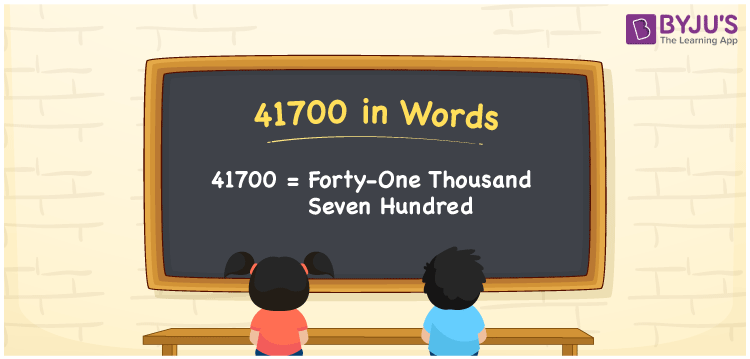# 41700 in words

41700 in words is written as Forty One Thousand Seven Hundred. In 41700, 4 has a place value of ten thousand, 1 is in the place value of thousand and 7 is in the place value of hundred. The article on Place Value gives more information. The number 41700 is used in expressions that relate to money, distance, length, social media views, and many more. For example, “Can I buy a smart phone with Forty One Thousand Seven Hundred rupees?.”

 41700 in words Forty One Thousand Seven Hundred Forty One Thousand Seven Hundred in Numbers 41700

## 41700 in English Words## How to Write 41700 in Words?

We can convert 41700 to words using a place value chart. This can be done as follows. The number 41700 has 5 digits, so let’s make a chart that shows the place value up to 5 digits.

 Ten thousand Thousands Hundreds Tens Ones 4 1 7 0 0

Thus, we can write the expanded form as:

4 × Ten thousand + 1 × Thousand + 7 × Hundred + 0 × Ten + 0 × One

= 4 × 10000 + 1 × 1000 + 7 × 100 + 0 × 10 + 0 × 1

= 41700.

= Forty One Thousand Seven Hundred.

41700 is the natural number that is succeeded by 41699 and preceded by 41701.

41700 in words – Forty One Thousand Seven Hundred.

Is 41700 an odd number? – No.

Is 41700 an even number? – Yes.

Is 41700 a perfect square number? – No.

Is 41700 a perfect cube number? – No.

Is 41700 a prime number? – No.

Is 41700 a composite number? – Yes.

## Solved Example

1. Write the number 41700 in expanded form

Solution: 4 x 10000 + 1 x 1000 + 7 x 100 + 0 x 10 + 0 x 1

Or Just 4 x 10000 + 1 x 1000 + 7 x 100

We can write 41700 = 40000 + 1000 + 700 + 0 + 0

= 4 x 10000 + 1 x 1000 + 7 x 100 + 0 x 10 + 0 x 1.

## Frequently Asked Questions on 41700 in words

Q1

### How to write the number 41700 in words?

41700 in words is written as Forty One Thousand Seven Hundred.
Q2

### Is 41700 divisible by 3?

Yes. 41700 is divisible by 3.
Q3

### Is 41700 a prime number?

No. 41700 is not a prime number.